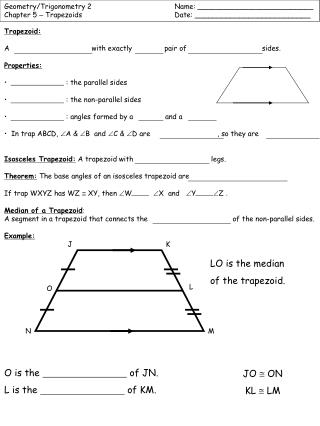# Trapezoid: A with exactly pair of sides. Properties: - PowerPoint PPT PresentationDownload PresentationTrapezoid: A with exactly pair of sides. Properties:

Trapezoid: A with exactly pair of sides. Properties:Download Presentation## Trapezoid: A with exactly pair of sides. Properties:

- - - - - - - - - - - - - - - - - - - - - - - - - - - E N D - - - - - - - - - - - - - - - - - - - - - - - - - - -
##### Presentation Transcript

1. Geometry/Trigonometry 2 Name: __________________________ Chapter 5 – Trapezoids Date: __________________________ J K L O N M • Trapezoid: • A with exactly pair of sides. • Properties: • : the parallel sides • : the non-parallel sides • : angles formed by a and a • In trap ABCD, A & B and C & D are , so they are • Isosceles Trapezoid: A trapezoid with legs. • Theorem: The base angles of an isosceles trapezoid are • If trap WXYZ has WZ  XY, then W X and Y Z . • Median of a Trapezoid: • A segment in a trapezoid that connects the of the non-parallel sides. • Example: LO is the median of the trapezoid. O is the of JN. L is the of KM. JO @ ON KL @ LM

2. Geometry/Trigonometry 2 Name: __________________________ Chapter 5 – Trapezoids Date: __________________________ 16 19 x 10 20 19 26 10 7x+3 6x + 10 15 x + 45 20 2x - 1 8x + 20 Property: The length of the median of a trapezoid is Examples: Sketch each trapezoid and complete the calculations for each median. 1.) 2.) 3.) 4.) 5.) 6.) x x x